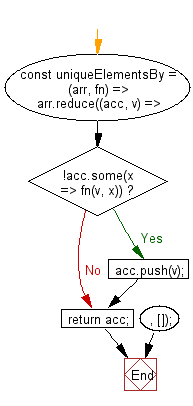# JavaScript: Get all unique values of an array, based on a provided comparator function

## JavaScript fundamental (ES6 Syntax): Exercise-108 with Solution

Write a JavaScript program to get all unique values of an array, based on a provided comparator function.

• Use Array.prototype.reduce() and Array.prototype.some() for an array containing only the first unique occurrence of each value, based on the comparator function, fn.
• The comparator function takes two arguments: the values of the two elements being compared.

Sample Solution:

JavaScript Code:

``````//#Source https://bit.ly/2neWfJ2
const uniqueElementsBy = (arr, fn) =>
arr.reduce((acc, v) => {
if (!acc.some(x => fn(v, x))) acc.push(v);
return acc;
}, []);

console.log(uniqueElementsBy(
[
{ id: 0, value: 'a' },
{ id: 1, value: 'b' },
{ id: 2, value: 'c' },
{ id: 1, value: 'd' },
{ id: 0, value: 'e' }
],
(a, b) => a.id == b.id
));
```
```

Sample Output:

```[{"id":0,"value":"a"},{"id":1,"value":"b"},{"id":2,"value":"c"}]
```

Flowchart:Live Demo:

See the Pen javascript-basic-exercise-108-1 by w3resource (@w3resource) on CodePen.

Improve this sample solution and post your code through Disqus

What is the difficulty level of this exercise?

Test your Programming skills with w3resource's quiz.

﻿

## JavaScript: Tips of the Day

Returns an array of n-tuples of consecutive elements

Example:

```const tips_arr = (n, arr) =>  n > arr.length ? [] : arr.slice(n - 1).map((v, i) =>[...arr.slice(i, i + n - 1), v]);
console.log(tips_arr(2, [1, 2, 3, 4, 5]));
console.log(tips_arr(3, [1, 2, 3, 4, 5]));
console.log(tips_arr(5, [1, 2, 3, 4]));
```

Output:

```[[1, 2], [2, 3], [3, 4], [4, 5]]
[[1, 2, 3], [2, 3, 4], [3, 4, 5]]
[]
```